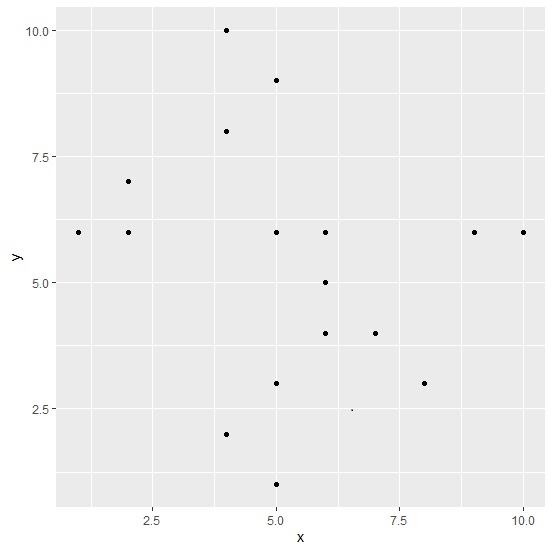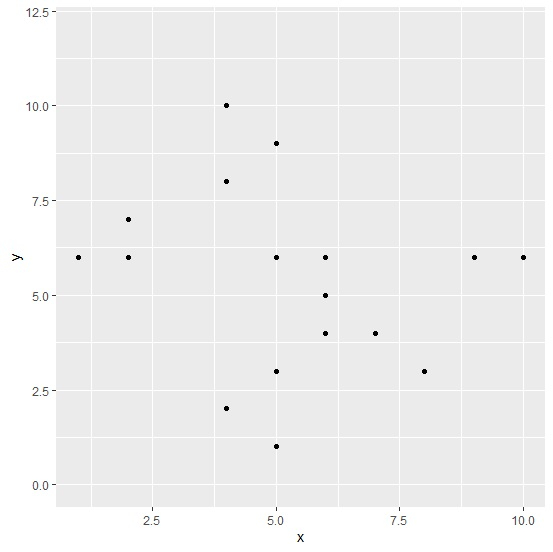# How to display 0 at Y-axis using ggplot2 in R?

To display 0 at Y-axis, we can set the limits for Y-axis using scale_y_continuous function of ggplot2 package. For example, if we have two columns say x and y in an R data frame called df then the scatterplot with displaying 0 at Y-axis can be created by using the below command

ggplot(df,aes(x,y))+geom_point()+scale_y_continuous(limits=c(0,”upperlimit”))

Consider the below data frame −

## Example

Live Demo

x<-rpois(20,5)
y<-rpois(20,5)
df<-data.frame(x,y)
df

## Output

   x  y
1  5  9
2  7  4
3  5  3
4  6  6
5  6  5
6  6  4
7  4  8
8  9  6
9  6  5
10 8  3
11 2  6
12 10 6
13 5  3
14 1  6
15 5  3
16 5  6
17 5  1
18 4  2
19 2  7
20 4  10

## Example

library(ggplot2)
ggplot(df,aes(x,y))+geom_point()

## OutputCreating the scatterplot of x and y with display of 0 on Y-axis −

## Example

ggplot(df,aes(x,y))+geom_point()+scale_y_continuous(limits=c(0,12))

## Output